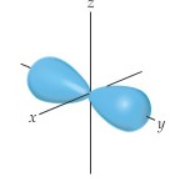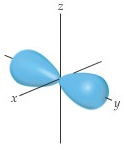# Problem: The contour representation of one of the orbitals for the n = 3 shell of a hydrogen atom is shown in the figure below.What is the quantum number ell for this orbital?

###### FREE Expert Solution

We are asked to identify the quantum number for this orbitalRecall that the quantum numbers that define an electron are:

• Principal Quantum Number (n): deals with the size and energy of the atomic orbital. The possible values for n are 1 to ∞.

• Angular Momentum Quantum Number (l): deals with the shape of the atomic orbital. The possible values for l are 0 to n – 1.

• Magnetic Quantum Number (ml): deals with the orientation of the atomic orbital in 3D space. The possible values for ml are the range of –l to +l.

• Spin Quantum Number (ms): deals with the spin of the electron. The possible values for ms are either –1/2 (spin down) or +1/2 (spin up).

86% (186 ratings)###### Problem Details

The contour representation of one of the orbitals for the n = 3 shell of a hydrogen atom is shown in the figure below.What is the quantum number for this orbital?

Frequently Asked Questions

What scientific concept do you need to know in order to solve this problem?

Our tutors have indicated that to solve this problem you will need to apply the Quantum Numbers: Angular Momentum Quantum Number concept. You can view video lessons to learn Quantum Numbers: Angular Momentum Quantum Number. Or if you need more Quantum Numbers: Angular Momentum Quantum Number practice, you can also practice Quantum Numbers: Angular Momentum Quantum Number practice problems.

What professor is this problem relevant for?

Based on our data, we think this problem is relevant for Professor Meier's class at UNG.